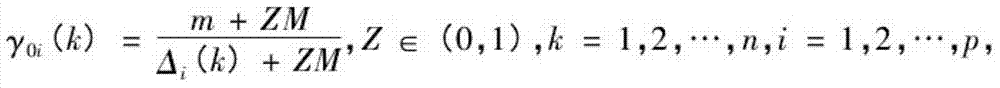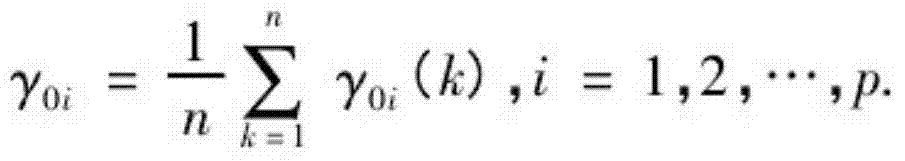# Grey rough set-based power grid construction project post-evaluation index weight assignment method

## A construction project, rough set technology, applied in the field of power grid construction evaluation, can solve the problem of no unified measurement standard

Inactive Publication Date: 2014-04-02
STATE GRID CORP OF CHINA +1
1 Cites 14 Cited by

## AI-Extracted Technical Summary

### Problems solved by technology

 Its disadvantage is that in the comprehensive evaluation, there is generally no unified measurement standard among the various characteristic ind...
View more

## Abstract

The invention discloses a grey rough set-based power grid construction project post-evaluation index weight assignment method, which comprises the following steps of constructing an index system, namely establishing a power grid construction project post-evaluation index system with a hierarchical structure; solving the importance of each index, namely solving the importance of each index by adopting a grey rough set related principle; gradually constructing a sample space based on the post-evaluation index system by using a marking result of each expert as original sample data; calculating a rough set of each index by utilizing a weight value of each expert and a grey rough set method; assigning weights by using a normalization processing method; performing normalization calculation on the rough set, calculated by the grey rough set method, of the index of each level to obtain an objective weight value of each index. According to the grey rough set-based power grid construction project post-evaluation index weight assignment method, the weight of the power grid construction project post-evaluation index of each level is obtained, technical support is provided for the development of power grid construction project post-evaluation work in the future, and the post-evaluation index system is normalized.

Application Domain

Data processing applicationsInformation technology support system

Technology Topic

Technical supportWeight value +8

## Image

•••## Examples

• Experimental program(1)

### Example Embodiment

 The following describes in detail the gray rough set-based power grid construction project post-evaluation index weighting method provided by the present invention with reference to the accompanying drawings and specific embodiments.
 Such as figure 1 As shown, the gray rough set-based power grid construction project post-evaluation index weighting method provided by the present invention includes the following steps executed in sequence:
 Step 1. The S01 stage of constructing the index system: First, establish a hierarchical structure of the grid construction project post-evaluation index system;
 According to the content of the post-evaluation, four first-level indicators are established from four aspects: management evaluation, financial evaluation, technical evaluation, and social impact evaluation. In order to fully evaluate a certain first-level indicator, you can Next, establish a number of secondary indicators and their evaluation weights according to the needs of the evaluation.
 Project implementation management evaluation mainly includes the evaluation of secondary indicators such as preliminary project evaluation, construction implementation evaluation, investment control evaluation, and basic management evaluation.
 The technical evaluation of power grid construction projects should fully consider the current status and interrelationship of the power grid, and establish a specific and practical technical evaluation index system for the types and characteristics of post-evaluation projects for evaluation. Technical evaluation secondary evaluation indicators generally include grid structure layout, power supply reliability, voltage quality, power supply capacity, etc.
 The main work of the financial evaluation of power grid construction projects is to analyze the profitability and solvency, focusing on the economic evaluation of the input and output of regional power grid construction.
 Project social impact evaluation is to analyze the contribution and impact of the evaluation object to the realization of various social development goals in the area and the adaptability to the society. The purpose is to adapt and coordinate the project with social development, to ensure the smooth implementation of the project and its sustained benefits. Enhance the beneficial effects of the project, reduce or eliminate the adverse effects, and promote social progress and development. It mainly includes four secondary indicators: environmental impact assessment, impact on residents' lives, impact on regional economy, and benefit evaluation of stakeholders.
 In order to further demonstrate the relationship between the post-evaluation index system and actual engineering projects and ensure the operability of post-evaluation work, it is necessary to continue to decompose each secondary index into three or four three-level indicators based on the analytic hierarchy process.
 Step 2: Solve the importance of each index in the S02 stage: use the gray rough set related principles to solve the importance of each index, that is, use the gray rough set theory to calculate the rough set of each index and solve the importance of each index ; Take the scoring results of each expert as the original sample data, and construct the sample space successively based on the above-mentioned post-evaluation index system hierarchical level; adopt the weight value of each expert, and use the gray rough set method to calculate the rough set of each index;
 Step 3. Use the normalization processing method to assign the weight S03 stage: according to the importance of each index, use the normalization processing method to assign the weight, that is, according to the importance of each index, use the normalization processing method to solve each Index weight: normalize the rough set of each level index calculated by the gray rough set method to obtain the objective weight value of each index.
 In the S01 stage, the post-evaluation index system of the power grid construction project is as follows:

 In the S02 stage, the gray rough set theory is used to calculate the rough set of each indicator, that is, the gray rough set method is used to calculate the objective weight value of each indicator, and the method of normalization after calculating the composite value of a single indicator includes Follow these steps in order:
 Step 1: The S201 stage of preprocessing the original data, establish the sample space set,
 X i =(X i (1), X i (2),……,X i (n)), i=0, 1, ..., p;
 Step 2: S202 phase of the difference sequence;
 Δ i (k)=|X 0 (k)-X i (k)|
 Δ i =(Δ i (1), Δ i (2),……,Δ i (n)), i=0, 1, ..., p;
 Step 3: Find the S203 stage of the two-level maximum difference and minimum difference;
 M=max i max k (k)
 m=min i min k (k)
 Step 4: Calculate the correlation coefficient to obtain the S204 stage of rough set;
 γ 0 i ( k ) = m + ZM Δ i ( k ) + ZM , Z A ( 0,1 ) , k = 1,2 , . . . , n , i = 1,2 , . . . , p ,
 Among them: Z is the resolution coefficient, its value only affects the size of the correlation coefficient, does not affect the degree of correlation, generally 0.5.
 Find the degree of relevance:
 γ 0 i = 1 n X k = 1 n γ 0 i ( k ) , i = 1,2 , . . . , p .
 The correlation degree of each attribute is γ(a 1 ), γ(a 2 ),..., γ(a m ).
 In the S03 stage, the normalization processing method is to substitute the rough set of each index into the normalization processing formula:
 w k - r k X k = 1 n r k
 Therefore, the weight value of the first-level indicator can be obtained;
 From the above calculation steps, the correlation degree of each attribute is γ(a 1 ), γ(a 2 ),..., γ(a m ), normalize it, and use the degree of association as a measure of attribute weight to obtain the attribute weight:
 η i = γ ( a i ) X j = 1 m γ ( a j ) , And X i = 1 m η i = 1 . .
 The gray rough set-based power grid construction project post-evaluation index weighting method provided by the present invention realizes the transformation from qualitative analysis to quantitative analysis of the power grid construction project post-evaluation indicators, and the gray rough set weighting method is adopted to obtain the weight of each indicator; Post-evaluation indicators for power grid construction projects include implementation management evaluation, financial evaluation, technical evaluation, and social impact evaluation.
 The following specifically describes the calculation method of the weight value of the first-level index, the calculation process of other second-level and third-level indicators is similar;
 The gray rough set method is used to calculate the objective weight value of each indicator, and the composite value of a single indicator is calculated and then normalized; take the first-level indicator processing process as an example:

 Step 1: Preprocess the original data to establish a sample space set. X i =(X i (1), X i (2),……,X i (n)),=0,1,……,p
 Here,
 X 0 =(0.4, 0.3, 0.3, 0.35, 0.4, 0.3, 0.2, 0.3)
 X 1 =(0.3, 0.3, 0.1, 0.35, 0.4, 0.3, 0.3, 0.25)
 X 2 =(0.2, 0.3, 0.3, 0.2, 0.1, 0.3, 0.3, 0.25)
 X 3 =(0.1, 0.1, 0.3, 0.1, 0.1, 0.1, 0.2, 0.2)
 Step two, find the difference sequence,
 Δ i (k)=|X 0 (k)-X i (k)|
 Δ i =(Δ i (1), Δ i (2),……,Δ i (n)), i=0, 1, ..., p
 Δ = 0 0 0 0 0 0 0 0 0.1 0 0.2 0 0 0 0.1 0.05 0.2 0 0 0.15 0.3 0 0.1 0.05 0.3 0.2 0 0.25 0.3 0.2 0 0.1
 Step 3: Find the maximum difference and minimum difference of the two levels,
 M=max i max k (k)
 m=min i min k (k)
 M=(0.3 0.2 0.2 0.25 0.3 0.2 0.1 0.1)
 m=(0.1 0 0 0 0 0 0 0.05)
 Step 4: Calculate the correlation coefficient and obtain the rough set.
 γ 0 i ( k ) = m + ZM Δ i ( k ) + ZM , Z A ( 0,1 ) , k = 1,2 , . . . , n , i = 1,2 , . . . , p ,
 γ 0 i = 1 n X k = 1 n γ 0 i ( k ) , i = 1,2 , . . . , p .
 γ = 1.67 1 1 1 1 1 1 2 1 1 0.33 1 1 1 0.33 1 0.71 1 1 0.45 0.33 1 0.33 1 0.56 0.33 1 0.33 0.33 0.33 1 0.67
 η=(1.21 0.83 0.73 0.57)
 Step 5: Normalize the rough set of each indicator, the calculation formula is:
 w k = r k X k = 1 n r k
 w=(0.36 0.25 0.22 0.17)
 Therefore, the weight value of the first-level index can be obtained.

## PUM## Description & Claims & Application Information

We can also present the details of the Description, Claims and Application information to help users get a comprehensive understanding of the technical details of the patent, such as background art, summary of invention, brief description of drawings, description of embodiments, and other original content. On the other hand, users can also determine the specific scope of protection of the technology through the list of claims; as well as understand the changes in the life cycle of the technology with the presentation of the patent timeline. Login to view more.
Who we serve
• R&D Engineer
• R&D Manager
• IP Professional
Why Eureka